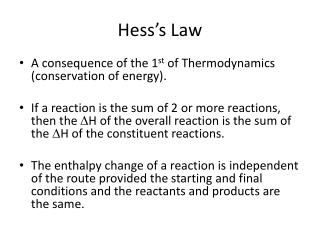DownloadDownload PresentationHess’s Law

# Hess’s Law

Télécharger la présentation## Hess’s Law

- - - - - - - - - - - - - - - - - - - - - - - - - - - E N D - - - - - - - - - - - - - - - - - - - - - - - - - - -
##### Presentation Transcript

1. Hess’s Law A consequence of the 1st of Thermodynamics (conservation of energy). If a reaction is the sum of 2 or more reactions, then the H of the overall reaction is the sum of the H of the constituent reactions. The enthalpy change of a reaction is independent of the route provided the starting and final conditions and the reactants and products are the same.
2. Example 1 From the information below, calculateH, for the reaction CO (g) + ½ O2(g)  CO2(g) C (s) + O2 (g) CO2 (g) H = -393 kJ C (s) + ½O2 (g)  CO(g)H = -283 kJ
3. Answer to example 1 C (s) + O2 (g)  CO2 (g) H = -393 kJ CO (g)  C (s) + ½ O2 (g) H = +283 kJ CO (g) + ½ O2 (g)  CO2 (g) H = -110 kJ Draw an enthalpy level diagram and an enthalpy cycle for this example.
4. Drawing and energy cycle Use the frameworks below to build your cycle. Usually the equation of the unknown enthalpy change is the horizontal equation. Either all three arrows represent equations and therefore each equation should be balanced. Or all particles at each of the three points are equal; show them in boxes. Use arrows to write a formula to calculate unknown enthalpy change.
5. Example 2 From the information below, calculateH, for the reaction S (s) + O2(g)  SO2(g) SO2(g) + ½ O2(g) SO3 (g) H = - 98 kJ S (s) + 1 ½ O2 (g)  SO3 (g)H = -395 kJ
6. Answer to example 2 S (s) + 1 ½ O2 (g)  SO3 (g) H = -395 kJ SO3 (g)  SO2 (g) + ½ O2 (g) H = +98 kJ S (s) + O2 (g)  SO2 (g) H = -297 kJ Draw an enthalpy level diagram and an enthalpy cycle for this example.
7. Example 3 Calculate the H for CaCO3(s) CaO (s) + CO2 (g) From the following equations and enthalpy changes: CaCO3(s) + 2HCl (aq)  CaCl2 (aq) + CO2 (g)+ H2O (l) H= -17 kJ mol-1 CaO(s) + 2HCl (aq)  CaCl2 (aq) + H2O (l) H= -195 kJ mol-1
8. Answer example 3 CaCO3(s) + 2HCl (aq)  CaCl2 (aq) + CO2 (g)+ H2O (l) H= -17 kJ mol-1 CaCl2 (aq) + H2O (l) CaO(s) + 2HCl (aq) H= + 195 kJ mol-1 CaCO3CaO (s) + CO2 (g) H= +178 kJ mol-1
9. Example 4 Calculate C(s) + 2H2 (g)  CH4 (g) H = ? kJ when given the following CH4(g) + 2 O2(g)  CO2 (g) + 2H2O(l) H = - 890.4 kJ C (s) + O2 (g)  CO2 (g) H = - 393.4 kJ 2 H2 (g) + O2 (g)  2 H2O (l) H = - 571.6 kJ
10. Answer example 4 Reverse the first equation but leave the next two as they are CO2(g) + 2H2O (l)  CH4 (g) + 2O2 (g) H = + 890.4 kJ C (s) + O2 (g)  CO2 (g) H = - 393.4 kJ 2 H2 (g) + O2 (g) 2H2O (l) H = - 571.6 kJ C(s) + 2H2(g)  CH4 (g)H = - 74.7 kJ
11. Example 5 Calculate Hf for ICl (g) the following ½ I2 (s) + ½ Cl2 (g) ICl (g) when given the following Cl2(g)  2 Cl (g) H = + 242 kJ I2(g)  2 I (g) H = + 151 kJ I2(s)  I2 (g) H = + 63 kJ ICl (g) Cl (g) + I (g) H = + 211 kJ
12. Answer example 5 Solution: take half a mole for each of the first equations and reverse the fourth. ½ Cl2 (g) Cl (g) H = + 121 kJ ½ I2 (g)  I (g) H = + 75.5 kJ ½ I2 (s)  ½ I2 (g) H = + 31.5 kJ Cl(g) + I (g) ICl (g) H = - 211 kJ ½ I2 (s) + ½ Cl2 (g) ICl (g) H = + 17 kJ